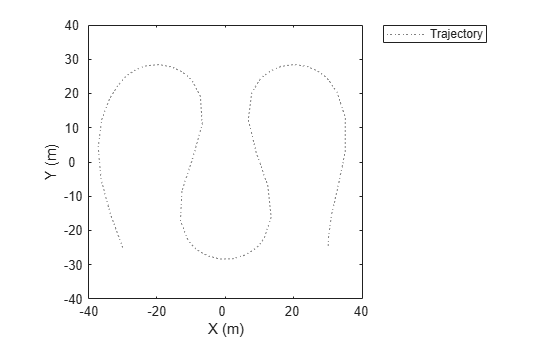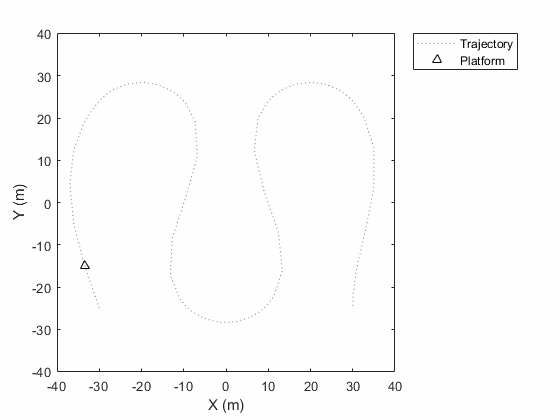plotTrajectory

Plot set of trajectories in trajectory plotter

Description

example

plotTrajectory(trajPlotter,trajCoordList) specifies the trajectories to show in the trajectory plotter, trajPlotter. The input argument trajCoordList is a cell array of M-by-3 matrices, where M is the number of points in the trajectory. Each matrix in trajCoordList can have a different number of rows. The first, second, and third columns of each matrix correspond to the x-, y-, and z-coordinates of a curve through M points that represent the corresponding trajectory.

Examples

collapse all

This example shows how to create an animation of a platform moving on a trajectory.

height = 100;
d = 1;
wayPoints = [ ...
-30   -25   height;
-30    25-d height;
-30+d  25   height;
-10-d  25   height;
-10    25-d height;
-10   -25+d height;
-10+d -25   height;
10-d -25   height;
10   -25+d height;
10    25-d height;
10+d  25   height;
30-d  25   height;
30    25-d height;
30   -25+d height;
30   -25   height];

Specify a time for each waypoint.

elapsedTime = linspace(0,10,size(wayPoints,1));

Next, create a platform in the tracking scenario and add trajectory information using the trajectory method.

target = platform(ts);
traj = waypointTrajectory('Waypoints',wayPoints,'TimeOfArrival',elapsedTime);
target.Trajectory = traj;

Record the tracking scenario to retrieve the platform's trajectory.

r = record(ts);
pposes = [r(:).Poses];
pposition = vertcat(pposes.Position);

Create a theater plot to display the recorded trajectory.

tp = theaterPlot('XLim',[-40 40],'YLim',[-40 40]);
trajPlotter = trajectoryPlotter(tp,'DisplayName','Trajectory');
plotTrajectory(trajPlotter,{pposition})Animate using the platformPlotter.

restart(ts);
trajPlotter = platformPlotter(tp,'DisplayName','Platform');

p = pose(target,'true');
plotPlatform(trajPlotter, p.Position);
pause(0.1)

endThis animation loops through all the generated plots.Input Arguments

collapse all

Trajectory plotter, specified as a trajectoryPlotter object.

Coordinates of trajectories to show, specified as a cell array of M-by-3 matrices, where M is the number of points in the trajectory. Each matrix in trajCoordList can have a different number of rows. The first, second, and third columns of each matrix correspond to the x-, y-, and z-coordinates of a curve through M points that represent the corresponding trajectory.

Example: coordList = {[1 2 3; 4 5 6; 7,8,9];[4 2 1; 4 3 1];[4 4 4; 3 1 2; 9 9 9; 1 0 2]} specifies three different trajectories.